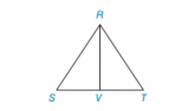Chapter 2.4, Problem 30E### Elementary Geometry for College St...

6th Edition
Daniel C. Alexander + 1 other
ISBN: 9781285195698

#### Solutions

Chapter
Section### Elementary Geometry for College St...

6th Edition
Daniel C. Alexander + 1 other
ISBN: 9781285195698
Textbook Problem
20 views

# Given: Equiangular Δ R S T Prove: R V → bisects ∠ S R T Δ R V S is a right ΔTo determine

To prove:

ΔRVS is a right angle triangle or not.

Explanation

Given:

Equiangular ΔRST and RV¯ bisects ΔSRT.

Figure (1)

Approach:

The sum of interior angles of a triangle is equal to 180°.

Calculation:

mS+mT+mSRT=180°

mS+mSRV+mRVS=180°

Since, bisects SRT. Therefore,

mSRV=12(mSRT)

Since, ΔRST is an equiangular triangle. Therefore,

mS=mT

Consider, mS+mSRV

Substitute 12(mSRT) for mSRV, mT for mS, and 12(2mS) for mT.

### Still sussing out bartleby?

Check out a sample textbook solution.

See a sample solution

#### The Solution to Your Study Problems

Bartleby provides explanations to thousands of textbook problems written by our experts, many with advanced degrees!

Get Started

#### Sketch the graphs of the equations in Exercises 512. xy=4

Finite Mathematics and Applied Calculus (MindTap Course List)

#### Find the limit or show that it does not exist. limx(x2+1)

Single Variable Calculus: Early Transcendentals, Volume I

#### 0 1 π It does not exist.

Study Guide for Stewart's Multivariable Calculus, 8th